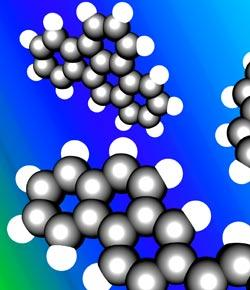# Unit 8 - Chemical Solutions Test

31 Questions | Total Attempts: 268SettingsChemical solutions are homogenous mixtures composed of two or more substances. This usually involves a solute being dissolved into another. Think you know all there is to know about chemical solutions? Try your hand at this scientific quiz!

• 1.
In a solution, the substance that is being dissolved is the _____.
• A.

Solute

• B.

Solvent

• C.

Liquid

• D.

Gas

• 2.
The air that you breathe is an example of a(n) ____.
• A.

Gas

• B.

Solid

• C.

Liquid

• D.

Amalgam

• 3.
In soda pop, the solvent would be the _____.
• A.

Water

• B.

Carbon dioxide

• C.

Sugar

• D.

Flavoring

• 4.
A substance that does not conduct an electric current when it forms a solution is a(n) _____.
• A.

Electrolyte

• B.

Nonelectrolyte

• C.

Polar substance

• D.

Salt

• 5.
A solution that contains all of the solute it can normally hold at a given temperature is _____.
• A.

Dilute

• B.

Unsaturated

• C.

Supersaturated

• D.

Saturated

• 6.
The process by which the particles in a crystal are separated and drawn into solution by water is called _____.
• A.

Ionization

• B.

Dissociation

• C.

Polarity

• D.

Saturation

• 7.
Increasing the surface area of a solid _____.
• A.

Slows the speed of dissolving

• B.

Has no effect on the speed of dissolving

• C.

Increases the speed of dissolving

• D.

Causes the solid to ionize

• 8.
The solubility of gas in a liquid occurs faster if the liquid is ______.
• A.

Heated

• B.

Cold

• C.

Under low pressure

• D.

An electrolyte

• 9.
Which of the the following types of solutes dissolves well in water?
• A.

Hydrocarbons

• B.

Polar molecules

• C.

Nonpolar molecules

• D.

Oil

• 10.
The concentration of a solution that contains a large amount of solute in the solvent could be described as _____.
• A.

Unsaturated

• B.

Polar

• C.

Concentrated

• D.

Dilute

• 11.
Which of the following would dissolve in iodine?
• A.

Water

• B.

Ethanol

• C.

Polar solvent

• D.

Vinegar

• 12.
An alloy is an example of a _____ solution.
• A.

Gaseous

• B.

Liquid

• C.

Solid

• D.

Dilute

• 13.
Adding more solute to a solvent _____.
• A.

Raises its freezing point

• B.

Lowers its boiling point

• C.

Raises its boiling point

• D.

Does not affect its boiling point

• 14.
What is the first step of the dissolving process?
• A.

The molecules of the solvent pull the molecules of the solute into solution.

• B.

The molecules of the solute pull the molecules of the solvent into solution.

• C.

The moving molecules of the solvent spread the molecules of the solute equally throughout the solution.

• D.

The moving molecules of the solvent surround the molecules of the solute.

• 15.
Which of the following combination would increase the solubility of a gas in a liquid?
• A.

Increase the pressure and decrease the temperature

• B.

Increase the pressure and temperature

• C.

Decrease the pressure and temperature

• D.

Decrease the pressure and increase the temperature

• 16.
Which of the following will speed up the dissolving of a solid solute in water?
• A.

Stir the solution

• B.

Cool the solution

• C.

Grind up the solvent

• D.

Freeze the solute

• 17.
Water is sometimes referred to as the universal solvent because _____.
• A.

It has no separated positive and negative areas

• B.

It is a small molecule and can fit easily among the molecules of many solutes

• C.

It has a polar and nonpolar end

• D.

Its molecules can slip easily among molecules of a nonpolar solvent

• 18.
One commonly used precise way to describe concentrations of solutions is to state _____.
• A.

That the solution is dilute or concentrated

• B.

The percentage by area of the solute

• C.

The percentage by volume of the solute

• D.

The percentage by volume of the solvent

• 19.
A molecule with a positive and negative area is ___.
• A.

Polar

• B.

Nonpolar

• C.

Ionic

• D.

• 20.
The maximum amount of a solute that can be dissolved in a given amount of solvent is its ______.
• A.

Concentration

• B.

Density

• C.

Solubility

• D.

Dilution

• 21.
Soap has a(n) ______ end that will dissolve in water and has a long hydrocarbon proton that will dissolve in oily dirt.
• A.

Ethanol

• B.

Dry

• C.

Toxic

• D.

Ionic

• 22.
Adding a solute to a solvent raises the freezing point of the solvent.
• A.

True

• B.

False

• 23.
Adding a solute to a solvent raises the boiling point of a solvent.
• A.

True

• B.

False

• 24.
A substance that produces ions in water is a nonelectrolyte.
• A.

True

• B.

False

• 25.
The ocean is a solution because it is a mixture of salts and water.
• A.

True

• B.

False

Related TopicsBack to top